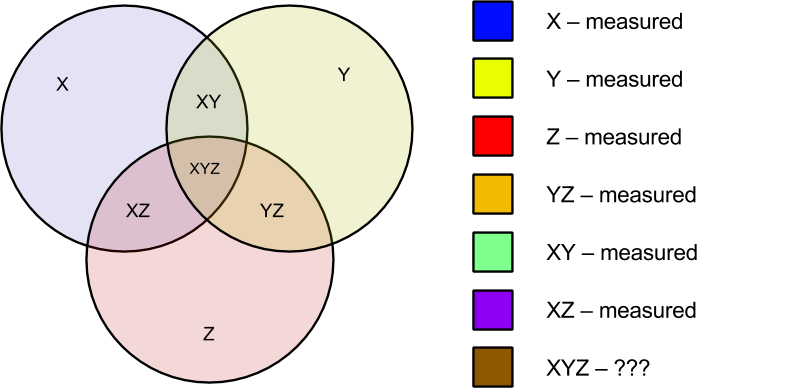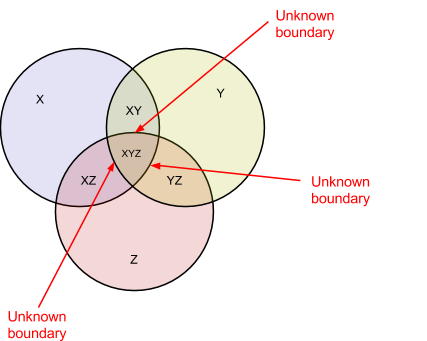# One Big Fluke

I'm Brett Slatkin and this is where I write about programming and related topics. You can contact me here or view my projects.

## 18 April 2014

### Data fusion has no error bounds

Over the years I've seen attempts to solve what is called the "data fusion" problem. What is that? You have one useful dataset. You have another useful dataset. The goal is to somehow merge them together to create one larger, unified, and more powerful dataset. Sounds awesome! The problem is the two datasets are disjoint and thus have no overlapping sources. There is no simple key with which to join them together.

Companies have been built and busted trying to accomplish this, often in the advertising space. The same idea applies to likely-voter modeling and more.

So can it be done? Let me show you with a simple example.

Imagine you have 3 variables and you want to measure their correlation: X, Y, and Z. For this example let's say X is owning a car, Y is playing golf, and Z is traveling every week for work. Our hypothesis is these have a high correlation.

Say you send 3 separate surveys, A, B, and C, to different groups of random people to measure these variables.

Survey A is like this (X and Y):

Q1. Do you drive a car? [Yes | No]
Q2. Do you play golf? [Yes | No]

Survey B is like this (Y and Z):

Q1. Do you play golf? [Yes | No]
Q2. Do you travel weekly for work? [Yes | No]

Survey C is like this (X and Z):

Q1. Do you drive a car? [Yes | No]
Q2. Do you travel weekly for work? [Yes | No]

Survey A gives you correlation of Car and Golf, variables X and Y. Survey B gives you correlation of Golf and Travel, variables Y and Z. Survey C gives you correlation of Car and Travel, variables X and Z. That leads to this question:

With datasets for correlation of XY, correlation of YZ, and correlation of XZ, can you calculate the correlation of XYZ? This is exactly data fusion problem. The answer is:

No, you can't. Here's why:You haven't measured XYZ. How do you calculate it? How can you put boundaries on its size? There are actually 8 set memberships you're trying to determine:You know none of these.

You could assume a uniform distribution of Z in the set XY. Assuming Z (Traveling) is split Yes/No as 40/60 in the general population (the red circle), then also assume it's split 40/60 in the Car & Golf population set (the green section, XY). That sounds reasonable, but there is no way to actually calculate an error boundary on that assumption. You have no idea what the interior of XYZ looks like. It could be a "rogue wave" of correlation, where the distribution of Z (Traveling) in the set XY (Car/Golf) is perfect and the correlation of XYZ is 100%. It could just as easily be the opposite, where the correlation of XYZ is 0%. You have no way of knowing. All of the data measurements you have collected cannot reveal any pieces of the XYZ interior.Thus, you must assume the error boundary on XYZ is 100%. There's no way to calculate otherwise. If you want to calculate XYZ, you must measure XYZ. No modeling or bias correction can compensate for this. There are two outcomes in data fusion: you measure so you can calculate the error bars, or you make a wild guess.
Contact